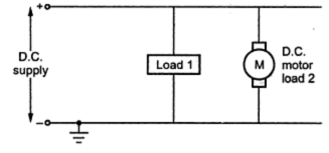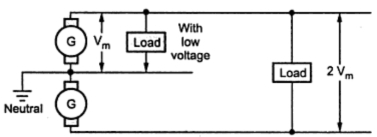### D.C. Systems

Though a.c. is extensively used everywhere, there are few applications like operation of d.c. motors, batteries, charging where d.c. supply is must. It can be obtained by using rectifiers or by d.c. generators at substations.
The d.c. systems are further classified as,
1. Two wire d.c. system
2. Two wire with midpoint earthed d.c. system
3. Three wire d.c. system
1.1 Two Wire D.C. System
It consists of two wires, one positive and other negative. The positive is outgoing while the negative is return wire. The two wire d.c. system where d.c. generators are used at the generating station is shown in the Fig. 1.Fig. 1 Scheme of two wire d.c. system

The symbolic representation of two wire d.c. system is shown in the Fig. 2. The various loads are connected in parallel.Fig. 2  Two wire d.c. system

The details like feeders, distributors and service mains are not included in the symbolic representation. The negative line is generally earthed.

1.2 Two Wire D.C. System with Midpoint Earthed
In this system, there are two line conductors, the one having voltage of + with respect to midpoint which is earthed while the other is at potential - with respect to midpoint. Hence the total voltage available between the two lines is 2V. No connection is taken out from the midpoint, which is earthed at the substation. The loads requiring high d.c. voltages are connected across the lines. The symbolic representation of this system is shown in the Fig. 3.Fig. 3 Two wire d.c. system with midpoint earthed

The volume of copper required in this system is less than that required in two wire d.c. system with one line earthed.

1.3 Three Wire D.C. System
This system is exactly similar to the two wire d.c. system with midpoint earthed. The only difference is, additional third wire from earth terminal of substation is available for the connections. The scheme is shown in the Fig. 4. This wire is also called neutral wire.Fig. 4 Three wire d.c. system

When the load is balanced, no current flows through the neutral.
The loads requiring higher voltages are connected across the two outers while the loads requiring  low voltages are connected between one outer and neutral as shown. The volume of copper required is higher than two wire midpoint earthed system but less than two wire one line earthed system.

The various advantages of using d.c. system for the transmission and distribution are,
1. As the frequency of d.c. is zero, there is no inductancen or capacitance associated with the line. Hence the power losses and voltage drops are much less compared to a.c. system.
2. Due to the reduced voltage drops, the voltage regulation is better.
3. Absence of skin effect makes use of entire cross-section of conductor.
4. For the same voltage level, the voltage stress on the insulation is less in a d.c. system. Hence the insulation required in case of d.c. is less compared to a.c. system.
5. As the stress on cable insulation is less, the economical use of undeground cables is possible.
6. The stability problems as well as synchronizing difficulties are absent in a d.c. system.
7. The d.c. line has reduced interference with radio and communication circuits.
8. In a three phase a.c. system. So copper requirement is less.